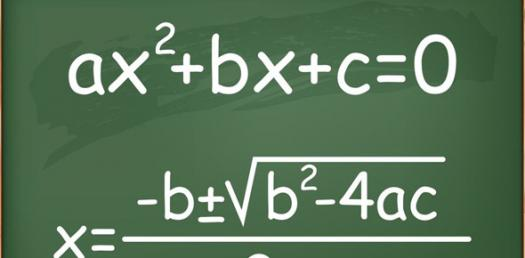Integer And Rational Exponents Quiz

10 Questions | Total Attempts: 944SettingsAlgebra 1 is a foundation book helping you get core skills. As you have now covered integers and rational exponents thoroughly in the past week you can now be ready to take the test next week. Take up the quiz below to gauge how ready you are. All the best!

• 1.
Which of the following is NOT an explanation of the rule for simplifying expressions containing a negative exponent?
• A.

Use the reciprocal of the base and change the exponent to a positive number.

• B.

Use the denominator of the exponent as a root of the base. Use the numerator as a power.

• C.

Use 1 divided by that base raised to the opposite (positive) exponent.

• D.

Make the exponent positive and move the factor to the other side of the fraction bar.

Related TopicsBack to top